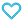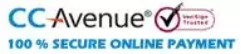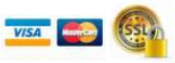# Handbook of Mathematics For JEE, CUET, Boards and Various Competitive Exams

250.00

• Paper Book

MTG launched Handbook for Mathematics that covers the syllabus for JEE, Boards and CUET. It is the most ideal handbook according to every aspirant’s requirements and is perfect for quick revisions. The Handbook is compact, concise and convenient to carry so that students can revise anywhere, anytime. The handbook covers crisp notes, terms, definitions, formulas, HD images and diagrams which makes it perfect for last-minute recaps and memorising formulas.

997 in stock

Qty:Add to WishlistMTG is introducing its first edition of the Handbook for Mathematics which will work as a revision module beneficial for revising the entire syllabus for NEET, JEE, Boards and CUET. MTG Handbook for Mathematics will help students with concept clarity at every step of the learning process. This book aims at providing precise content to students to make studying even the most difficult topics interactive and fun. This book gives every NEET/JEE/Board/CUET aspirant an opportunity to revise the subject while travelling or between two shifts of examination. This book can be conveniently carried around by students due to its compact design. Salient features of this book –

• NCERT insight – Short and crisp notes which cover all the NCERT content along with additional information to give an extra edge to your preparation.
• Terms and Definitions – All important terms and definitions of Mathematics.
• Tables and diagrams – For better understanding and concept clarity.
• Formulae, charts and graphs – For memorizing and a better understanding of concepts.

MTG handbook is curated by a team of competent, qualified and professional academicians to be the finest and error-free book. We hope that this book will be an excellent handbook and help you to face the exams with ease and confidence.

Table of Content:-
• Sets
• Relations
• Functions and Binary Operations
• Complex Numbers
• Sequences and Series
• Equations and Inequations
• Permutations and Combinations
• Principle of Mathematical Induction and Binomial Theorem
• Trigonometric Functions and Equations
• Application of Trigonometry
• Coordinate Geometry
• Straight Lines
• Circles
• Ellipse
• Hyperbola
• Inverse Trigonometric Functions
• Matrices
• Determinants
• Limits, Continuity and Differentiability
• Derivatives
• Application of Derivatives
• Integrals
• Application of Integrals
• Differential Equations
• Vectors
• Three Dimensional Geometry
• Mathematical Reasoning
• Linear Programming Problem
• Statistics
• Probability
• Elementary Arithmetic I
• Elementary Arithmetic II
• Elementary Arithmetic III
• Commercial Mathematics
• Logarithm
• Mensuration
• Appendix
ISBN11 9789355558671 MTG Editorial Board 2023-24 400 Class 12 CBSE Boards Mathematics 326gm